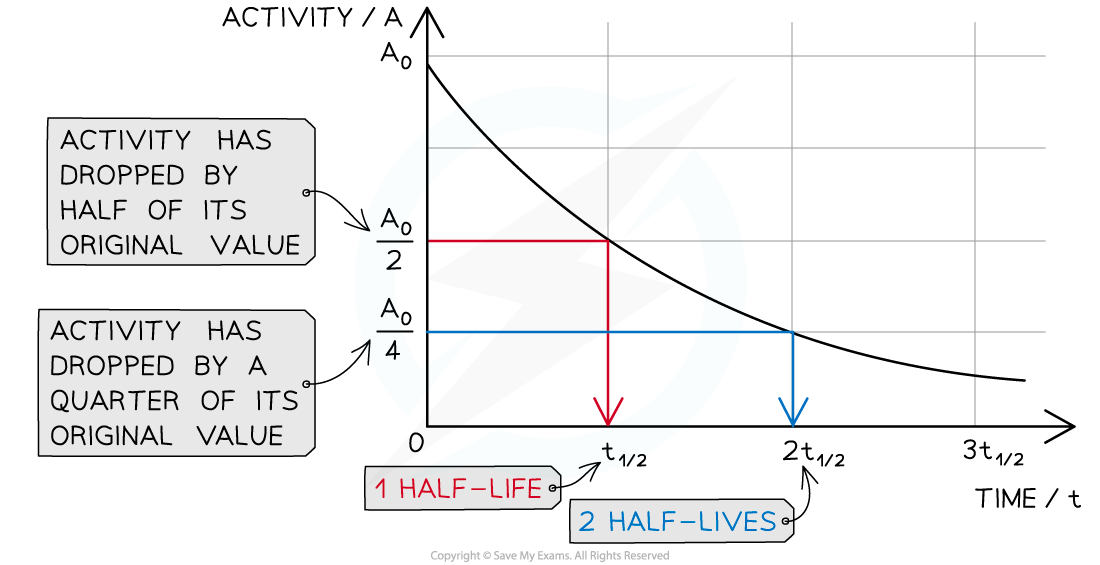# IB DP Physics: HL复习笔记7.1.8 Half-Life

### Half-Life

• It is impossible to know when a particular unstable nucleus will decay
• But the rate at which the activity of a sample decreases can be known
• This is known as the half-life
• Half-life is defined as:

The time taken for half the undecayed nuclei to decay or the activity of a source to decay by half

• In other words, the time it takes for the activity of a sample to fall to half its original level
• Different isotopes have different half-lives and half-lives can vary from a fraction of a second to billions of years in length

#### Using Half-life

• Scientists can measure the half-lives of different isotopes accurately:
• Uranium-235 has a half-life of 704 million years
• This means it would take 704 million years for the activity of a uranium-235 sample to decrease to half its original amount
• Carbon-14 has a half-life of 5700 years
• So after 5700 years, there would be 50% of the original amount of carbon-14 remaining
• After two half-lives, or 11 400 years, there would be just 25% of the carbon-14 remaining
• With each half-life, the amount remaining decreases by halfGraph showing how the activity of a radioactive sample changes over time. Each time the original activity halves, another half-life has passed

• The time it takes for the activity of the sample to decrease from 100 % to 50 % is the half-life
• It is the same length of time as it would take to decrease from 50 % activity to 25 % activity
• The half-life is constant for a particular isotope

### Decay Curves

• To calculate the half-life of a sample, the procedure is:
• Measure the initial activity, A0, of the sample
• Determine the half-life of this original activity
• Measure how the activity changes with time
• The time taken for the activity to decrease to half its original value is the half-life

#### Worked Example

The radioisotope technetium is used extensively in medicine. The graph below shows how the activity of a sample varies with time.Determine the half-life of this material.

Step 1: Draw lines on the graph to determine the time it takes for technetium to drop to half of its original activityStep 2: Read the half-life from the graph

• In the diagram above the initial activity, A0, is 8 × 107 Bq
• The time taken to decrease to 4 × 107 Bq, or ½A0, is 6 hours
• The time taken to decrease to 2 × 107 Bq is 6 more hours
• The time taken to decrease to 1 × 107 Bq is 6 more hours
• Therefore, the half-life of this isotope is 6 hours

#### Worked Example

A particular radioactive sample contains 2 million un-decayed atoms. After a year, there is only 500 000 atoms left un-decayed.

What is the half-life of this material?

Step 1: Calculate how many times the number of un-decayed atoms has halved

• 1 000 000 atoms would remain after 1 half-life
• 500 000 atoms would remain after 2 half-lives
• Therefore, the sample has undergone 2 half-lives

Step 2: Divide the time period by the number of half-lives

• The time period is a year
• The number of half-lives is 2
• 1 year divided by 2 is half a year or 6 months
• Therefore, the half-life is 6 months

#### Worked Example

A radioactive sample has a half-life of 3 years. What is the ratio of decayed : original nuclei, after 15 years?

Step 1: Calculate the number of half-lives

• The time period is 15 years
• The half-life is 3 years

15 ÷ 3 = 5

• There have been 5 half-lives

Step 2: Raise 1/2 to the number of half-lives

(1/2)5 = 1/32

• So 1/32 of the original nuclei are remaining

Step 3: Write the ratio correctly

• If 1/32 of the original nuclei are remaining, then 31/32 must have decayed
• Therefore, the ratio is 31 decayed : 32 remaining, or 31:32